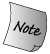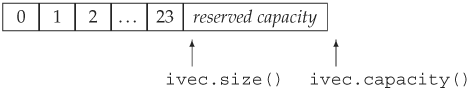### 9.4. vector 容器的自增长

When we insert or push an element onto a container object, the size of that object increases by one. Similarly, if we resize a container to be larger than its current size, then additional elements must be added to the container. The library takes care of allocating the memory to hold these new elements.

Ordinarily, we should not care about how a library type works: All we should care about is how to use it. However, in the case of vectors, a bit of the implementation leaks into its interface. To support fast random access, vector elements are stored contiguouslyeach element is adjacent to the previous element.

Given that elements are contiguous, let's think about what happens when we add an element to a vector: If there is no room in the vector for the new element, it cannot just add an element somewhere else in memory because the elements must be contiguous for indexing to work. Instead, the vector must allocate new memory to hold the existing elements plus the new one, copy the elements from the old location into the new space, add the new element, and deallocate the old memory. If vector did this memory allocation and deallocation each time we added an element, then performance would be unacceptably slow.

There is no comparable allocation issue for containers that do not hold their elements contiguously. For example, to add an element to a list, the library only needs to create the new element and chain it into the existing list. There is no need to reallocate or copy any of the existing elements.

One might conclude, therefore, that in general it is a good idea to use a list rather than a vector. However, the contrary is usually the case: For most applications the best container to use is a vector. The reason is that library implementors use allocation strategies that minimize the costs of storing elements contiguously. That cost is usually offset by the advantages in accessing elements that contiguous storage allows.

The way vectors achieve fast allocation is by allocating capacity beyond what is immediately needed. The vector holds this storage in reserve and uses it to allocate new elements as they are added. Thus, there is no need to reallocate the container for each new element. The exact amount of additional capacity allocated varies across different implementations of the library. This allocation strategy is dramatically more efficient than reallocating the container each time an element is added. In fact, its performance is good enough that in practice a vector usually grows more efficiently than a list or a deque.

#### 9.4.1. capacity 和 reserve 成员

The details of how vector handles its memory allocation are part of its implementation. However, a portion of this implementation is supported by the interface to vector. The vector class includes two members, capacity and reserve, that let us interact with the memory-allocation part of vector's implementation. The capacity operation tells us how many elements the container could hold before it must allocate more space. The reserve operation lets us tell the vector how many elements it should be prepared to hold.

vector 容器处理内存分配的细节是其实现的一部分。然而，该实现部分是由 vector 的接口支持的。vector 类提供了两个成员函数：capacityreserve 使程序员可与 vector 容器内存分配的实现部分交互工作。capacity 操作获取在容器需要分配更多的存储空间之前能够存储的元素总数，而 reserve 操作则告诉 vector 容器应该预留多少个元素的存储空间。It is important to understand the difference between capacity and size. The size is the number of elements in the vector; capacity is how many it could hold before new space must be allocated. 弄清楚容器的 capacity（容量）与 size（长度）的区别非常重要。size 指容器当前拥有的元素个数；而 capacity 则指容器在必须分配新存储空间之前可以存储的元素总数。

To illustrate the interaction between size and capacity, consider the following program:

```     vector<int> ivec;

// size should be zero; capacity is implementation defined
cout << "ivec: size: " << ivec.size()
<< " capacity: "  << ivec.capacity() << endl;

// give ivec 24 elements
for (vector<int>::size_type ix = 0; ix != 24; ++ix)
ivec.push_back(ix);

// size should be 24; capacity will be >= 24 and is implementation defined
cout << "ivec: size: " << ivec.size()
<< " capacity: "  << ivec.capacity() << endl;
```

When run on our system, this program produces the following output:

```     ivec: size: 0 capacity: 0
ivec: size: 24 capacity: 32
```

We know that the size of an empty vector is zero, and evidently our library also sets capacity of an empty vector to zero. When we add elements to the vector, we know that the size is the same as the number of elements we've added. The capacity must be at least as large as size but can be larger. Under this implementation, adding 24 elements one at a time results in a capacity of 32. Visually we can think of the current state of ivec asWe could now reserve some additional space:

```     ivec.reserve(50);  // sets capacity to at least 50; might be more
// size should be 24; capacity will be >= 50 and is implementation defined
cout << "ivec: size: " << ivec.size()
<< " capacity: "  << ivec.capacity() << endl;
```

As the output indicates, doing so changes the capacity but not the size:

```     ivec: size: 24 capacity: 50
```

We might next use up that reserved capacity as follows:

```     // add elements to use up the excess capacity
while (ivec.size() != ivec.capacity())
ivec.push_back(0);
// size should be 50; capacity should be unchanged
cout << "ivec: size: " << ivec.size()
<< " capacity: "  << ivec.capacity() << endl;
```

Because we used only reserved capacity, there is no need for the vector to do any allocation. In fact, as long as there is excess capacity, the vector must not reallocate its elements.

The output indicates that at this point we've used up the reserved capacity, and size and capacity are equal:

```     ivec: size: 50 capacity: 50
```

If we now add another element, the vector will have to reallocate itself:

```     ivec.push_back(42); // add one more element
// size should be 51; capacity will be >= 51 and is implementation defined
cout << "ivec: size: " << ivec.size()
<< " capacity: "  << ivec.capacity() << endl;
```

The output from this portion of the program

```     ivec: size: 51 capacity: 100
```

indicates that this vector implementation appears to follow a strategy of doubling the current capacity each time it has to allocate new storage.Each implementation of vector is free to choose its own allocation strategy. However, it must provide the reserve and capacity functions, and it must not allocate new memory until it is forced to do so. How much memory it allocates is up to the implementation. Different libraries will implement different strategies. vector 的每种实现都可自由地选择自己的内存分配策略。然而，它们都必须提供 vector 和 capacity 函数，而且必须是到必要时才分配新的内存空间。分配多少内存取决于其实现方式。不同的库采用不同的策略实现。

Moreover, every implementation is required to follow a strategy that ensures that it is efficient to use push_back to populate a vector. Technically speaking, the execution time of creating an n-element vector by calling push_back n times on an initially empty vector is never more than a constant multiple of n.

## Exercises Section 9.4.1

 Exercise 9.29: Explain the difference between a vector's capacity and its size. Why is it necessary to support the notion of capacity in a container that stores elements contiguously but not, for example, in a list? 解释 vector 的容量和长度之间的区别。为什么在连续存储元素的容器中需要支持“容量”的概念？而非连续的容器，如 list，则不需要。 Exercise 9.30: Write a program to explore the allocation stragegy followed by the library you use for vector objects. 编写程序研究标准库为 vector 对象提供的内存分配策略。 Exercise 9.31: Can a container have a capacity less than its size? Is a capacity equal to its size desirable? Initially? After an element is inserted? Why or why not? 容器的容量可以比其长度小吗？在初始时或插入元素后，容量是否恰好等于所需要的长度？为什么？ Exercise 9.32: Explain what the following program does: 解释下面程序实现的功能： ``` vector svec; svec.reserve(1024); string text_word; while (cin >> text_word) svec.push_back(text_word); svec.resize(svec.size()+svec.size()/2); ``` If the program reads 256 words, what is its likely capacity after it is resized? What if it reads 512? 1,000? 1,048? 如果该程序读入了 256 个单词，在调整大小后，该容器可能是多少？如果读入 512，或 1000，或 1048 个单词呢？